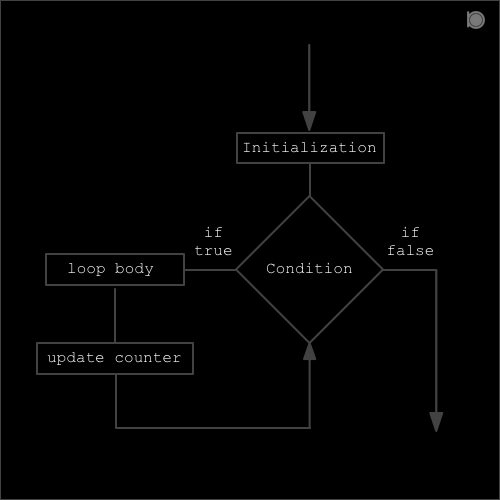for loop is a controlled loop which uses three expressions among which first is the initialization, second is the test condition and the last one is the counter updater.##### Syntax of for loop:
``````for(initialization; condition; inc/dec)
{
statements;
}
``````
1. First of all we need to initialize the counter variable.

2. Second step is to test our condition if the condition is true then the loop body is executed else the control from the loop will exit.

3. Third step is the execution of statements (loop body).

4. The last step is the updating the counter variable.

### Program example of for loop in C

``````//An example of for loop in C
#include<stdio.h>

int main()
{
for (int x = 0; x<10; x++)
{
printf("%d\n",x);
}
return 0;
}

``````

### output

``````0
1
2
3
4
5
6
7
8
9
``````